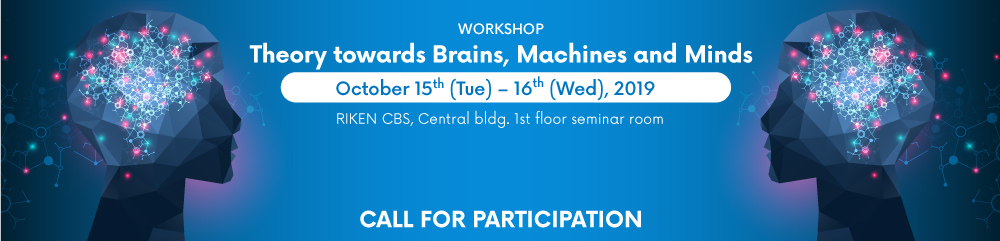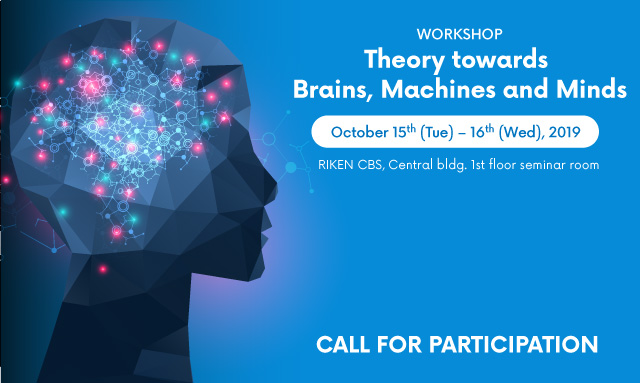## Title: An application of the drift diffusion model to the case of two-person binary choice problems

Mariko Ito, Graduate School of Information Science and Technology, The University of Tokyo

The drift diffusion model (DDM) describes agent's internal process of decision-making in two-alternative forced-choice problems. In this model, the internal state of an agent, who faces a binary choice problem between A and B, of which one is correct and the other is wrong, is modelled by a value called accumulated evidence, which follows a diffusion process. The model assumes that there are two boundaries. When the accumulated evidence hits the upper (the lower) boundary, it is regarded that this agent chooses A (B). This model helps us to discuss speed-accuracy trade-off in decision-making and has often been used for the interpretation of the results of psychological experiments. The correspondence between the value of accumulated evidence and the firing rate of some neurons has also been shown in neuroscience. Besides the importance of this model, the analytical expression of the probability distribution of the accumulated evidence has been poorly investigated.
Firstly, we derived the probability distribution of accumulated evidence analytically. Then we applied DDM to the case of two agents’ decision-making by using this analytical expression. Specifically, we assume that those two agents obey independent DDMs. We consider the situation in which one of the two agents hits a boundary, A or B, while the other has hit neither one yet. Then we ask what the optimal behavior by the latter agent is to maximize the probability of arriving at a correct choice, given the choice by the former agent is available to him/her. For that purpose, we assume that the accumulated evidence of the latter person can jump upwards (or downwards) when observing the former agent's choice, A or B. The width of the jump can be interpreted as the extent to which the latter person follows the former person. We find that the optimal jump width is always half of the difference between accumulated evidence of the self and that of the other if their initial evidence values are not biased. In other words, taking the average of two agents' accumulated evidence is always the best. It is known that taking average is a key to enjoying the effect of collective intelligence. In this presentation, we will discuss why we should assign the same weight on the opinions of two agents although the former agent has arrived at a conclusion earlier, by considering the effect of collective intelligence.